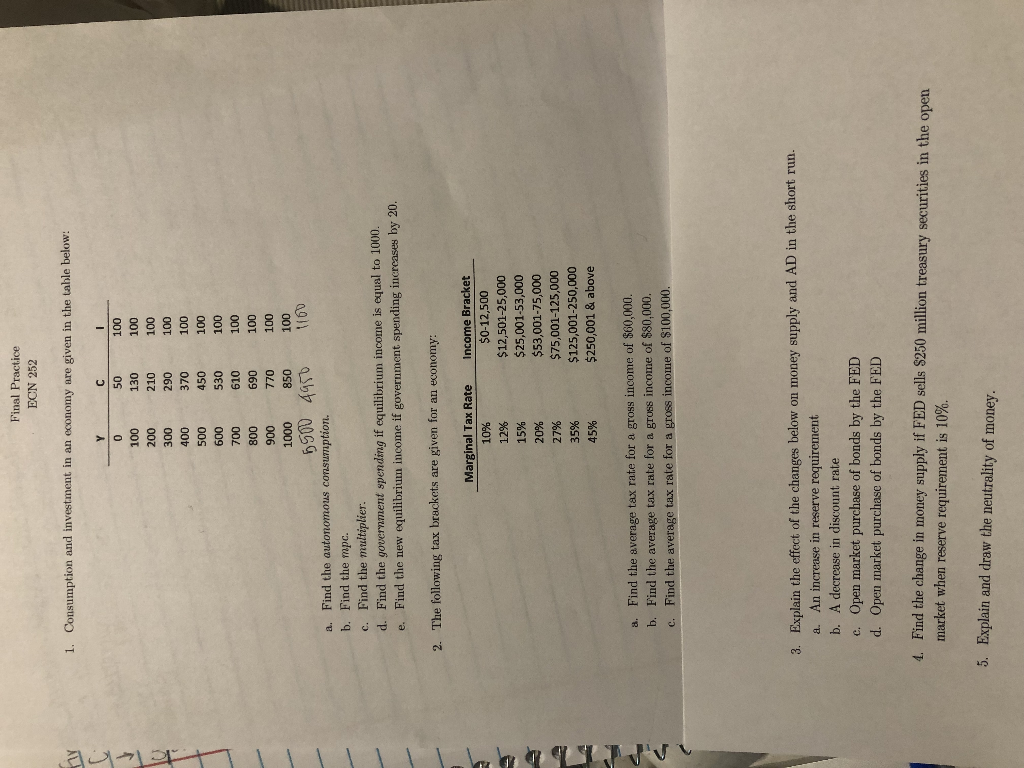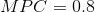Final Practice ECN 252 1. Consumption and investment in an economy are given in the table below: 0 0OT 001 002 00T 000 062 00T 00t 0LE 00T 00S 00T 530 009 001 00L OIS 00T 008 069 00T 0L 850 006 00T 000T 00T 0911 Find the autonomous consu7ption. b. Find the mpc. c. Find the multiplier. d. Find the government spending if equilibrium ncome is equal to 1000. Find the new equilibrium income if government spending increases by 20. The following tax brackets are given for an economy: Marginal Tax Rate Income Bracket 10% \$0-12,500 12 % \$12,501-25,000 \$25,001-53,000 \$53,001-75,000 15 % 27% \$75,001-125,000 \$125,001-250,000 \$250,001 & above 35% 45% a. Find the average tax rate for a gross income of \$60,000. b. Find the average tax rate for a gross income of \$80,000. Find the average tax rate for a gross income of \$100,000. 3. Explain the effect of the changes below on money supply and AD in the short run. a. An increase in reserve requirement b. A decrease in discount rate c. Open market purchase of bonds by the FED d. Open market purchase of bonds by the FED 4. Find the change in money supply if FED sells \$250 million treasury securities in the open market when reserve requirement is 10%. 5. Explain and draw the neutrality of money.

Chegg's guidelines ask an expert to answer the first four subparts of the first questions only. This is why the first 4 subparts are answered below.

a.

Autonomous consumption is the consumption at zero level of income. Therefore, autonomous consumption is of 50.

-------------------------------------------------------------------------------------------

b.

MPC is given as:Therefore, MPC is of 0.8.

---------------------------------------------------------------------------------------

c.

Multiplier is given as:Thus, multiplier is of 5.

---------------------------------------------------------------------------------------------------

d.

Government spending is calculated as:Thus, government spending increases by 200.

More Homework Help Questions Additional questions in this topic.